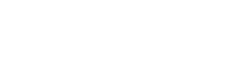# LCD Display Inverter

Display Inverter / VGA Board / LCD Controller

## Parameter definitions used in the IXYS power MOSFET datasheet

This application note describes the parameter definitions used in the IXYS power MOSFET datasheet. This document describes basic ratings and characteristics such as temperature, energy, mechanical data, and current and voltage ratings. It also briefly describes the graphics included in the data sheet and some equivalent diagrams for power MOSFETs.

This application note describes the parameter definitions used in the IXYS power MOSFET datasheet. This document describes basic ratings and characteristics such as temperature, energy, mechanical data, and current and voltage ratings. It also briefly describes the graphics included in the data sheet and some equivalent diagrams for power MOSFETs.

The parameters that IXYS provides for the datasheet are critical for selecting the right device and re-examining its performance in the application. The graphs included in the data sheets represent typical performance characteristics and can be used to extrapolate from one set of operating conditions to another. Power MOSFETs typically contain a body diode that provides “freewheeling” operation in inductive load switching. Figure 1 shows the equivalent circuits of N-channel and P-channel power MOSFETs.Figure 1 (a) an N-channel (b) a P-channel enhancement mode power MOSFET

Basic Ratings and Characteristics

top rating

Ratings are the limits of the device and are valid over the entire range of operating conditions.

temperature

Junction temperature TJ and TJM C In most cases, the range of junction temperature (TJ) is -55~150⁰C, which is the allowable temperature range where the device can operate continuously. The maximum junction temperature (TJM) is 150°C unless otherwise specified (175°C in some cases). The junction temperature changes the electrical parameters of the power MOSFET, for example, at very low temperatures (
Storage temperature TStg C is the temperature range in which the equipment is stored or transported and must be between -55~150⁰C

Lead Temperature TL C This is the maximum lead temperature during soldering and must not exceed 300⁰C for 10 seconds when 1/8″ from the case

Power consumption PD

Power dissipation is the maximum computational power the device can dissipate and is a function of thermal resistance at maximum junction temperature and case temperature TC25⁰C.current

Continuous On-State Drain Current ID25C This is the maximum current rating of the device at a case temperature (TC) of 25⁰C. It is calculated based on the maximum power dissipation, the maximum on-resistance and the temperature dependence of on-resistance. The current processing power of the leads may limit it.

Maximum Lead Current IDRMS C This is the maximum current rating of the device leads at a case temperature of 25°C.

Maximum Peak On-State Drain Current IDM C This is the peak current that the device can flow above the ID25 specification at the highest junction temperature. It varies with current pulse width, duty cycle and thermal conditions.

The diode forward current is C. This is the maximum DC current that the diode can flow in the forward direction at the specified case temperature.

Maximum Diode Forward Current ISM C This is the peak current that the diode can flow above the IS specification at the maximum junction temperature.

Voltage

Maximum Drain-Source Voltage VDSSS C The maximum drain-source voltage defines this without causing a gate-source short (VGS = 0) and avalanche breakdown occurs when the device is at 25⁰C. Avalanche breakdown voltage is temperature dependent and may be less than the BVDSS rating.

Maxim Gate-Source Voltage +/- VGS C This is the maximum voltage that can be introduced between gate and source. It depends on the thickness and characteristics of the gate oxide. The actual gate oxide withstand voltage is usually much higher than this value, but will vary due to the manufacturing process, so staying within this rating ensures application reliability.

The maximum rate of rise of the off-state voltage (dv/dt), C, is defined as the maximum allowable rate of rise of the off-state voltage across the device.

Avalanche energy (for avalanche equipment)

Avalanche Drain Current, Repetitive IAR C For power MOSFETs, the boom in current crowding in the chip area during an avalanche requires limiting avalanche current. It represents the avalanche energy specification of the device and the true capabilities of the device.

Maximum repetitive avalanche energy, maximum allowable reverse voltage breakdown energy for single-pulse EAR C continuous operation while respecting the maximum allowable die temperature. Heat dissipation limits avalanche energy.

Maximum non-repetitive avalanche energy, maximum allowable reverse voltage breakdown energy for EAS C continuous operation while respecting the maximum allowable die temperature. Heat dissipation limits avalanche energy.

Mechanical data

Mechanical Drawing C This provides packaging information for the mechanical dimensions of the device.

Weight C This provides weight information for the device with packaging.

Mounting torque Md C provides the maximum allowable mounting torque on the device.

Basic Curve DefinitionFigure 2 (a) an N-channel (b) a P-channel enhancement mode power MOSFET

Output characteristics

Figure 2 shows the typical output characteristics of an N-channel power MOSFET, where the different modes of operation are described. In the cut-off region, the gate-source voltage (VGS) is less than the gate threshold voltage (VGS(th)) and the device is in an open or off state. In the ohmic region, the device acts as a resistor whose on-resistance RDS(on) is almost constant and equal to VDS/IDS. In linear mode of operation, the device operates in the “current saturation” region where the drain current (Ids) is a function of the gate-source voltage (Vgs) and is defined by:where K is a temperature and device geometry dependent parameter and gfs is the current gain or transconductance. As the drain voltage (VDS) increases, the positive drain potential opposes the gate voltage bias and reduces the surface potential in the channel. The charge of the channel inversion layer decreases as VDS increases, and eventually, when the drain voltage is equal to (VGS-VGS(th)), the charge becomes zero. This point is called the “channel pinch point” at which the drain current becomes saturated.

The Links:   DMF50260NFU-FW-27 RM500HA-M